# Search PBS Space Time

## Results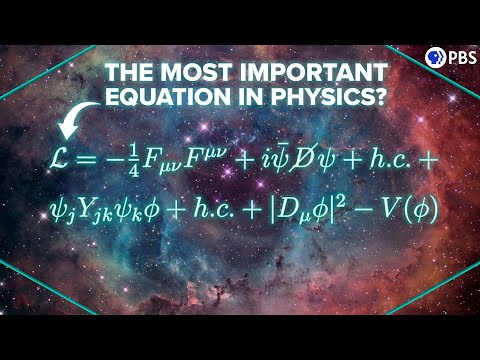### 2022-10-19: The Equation That Explains (Nearly) Everything!

• 01:02: ... One of the most important founding insights was the idea that the symmetries of nature give rise to the fundamental forces. Gauge invariance says ...
• 01:48: ... due to the fact that the universe has this particular rather simple symmetry - which we call a U(1) symmetry. That incredible success led many ...
• 02:21: ... symmetries also come from the fact that the wavefunction can be distorted in ...
• 04:05: ... motion, but Lagrangian is especially powerful because of how it respects symmetries. If the system that we are studying has a certain symmetry then the ...
• 04:36: ... a good idea to figure out the Lagrangian for a wavefunction that has our symmetries of interest. And that is exactly what the Standard Model Lagrangian is. ...
• 05:37: ... symmetries I mentioned allow the particles to have two different types of spins. ...
• 06:02: ... are called bosons. They transmit energy and momentum according to the symmetries of each force. Some parts of the standard model lagrangian deal with ...
• 06:57: ... represented with a capital A, this is the field that preserves the U(1) symmetry of electromagnetism, and the kinetic term for photons is made of the ...
• 08:37: ... many indices you see here come from the complex symmetries of SU(3), and account for all the different types of gluon. The bosons ...
• 09:45: ... us how fermions are gonna interact with the fields that preserve the symmetries of nature. This is the piece that tells us that matter and forces ...
• 10:36: ... charge, isospin, hypercharge, and color charge, and the more complex the symmetry the more indicies you need. Also, next to the charges are the coupling ...### 2022-08-24: What Makes The Strong Force Strong?

• 13:50: The reason this pattern arises in so many different places is a question of symmetry.
• 14:14: ... symmetry is baked into the laws of physics and there it manifests as the Strong ...
• 14:27: We have 3 colour receptors, and our brains use the SU(3) symmetry group to combine the input from those receptors into our subjective sense of colour.### 2022-07-20: What If We Live in a Superdeterministic Universe?

• 15:52: ... that have been chosen arbitrarily in order to break the underlying symmetries of ...
• 16:03: Agnibho Dutta asks if there’s any symmetry in physics, which holds for transformations in the color charge?
• 16:18: The symmetry in question is the strong force symmetry, known as the Special Unitary Group of order 3, or just SU(3).
• 17:56: ... it was a nefarious trick to teach you about hidden conventions and symmetries. ...### 2022-06-30: Could We Decode Alien Physics?

• 05:33: ... buried deep in the laws of physics is a subtle breaking of charge symmetry.  So in order to save the world from poorly assembled alien ...
• 07:38: ... a parity inversion. Parity is  another fundamental and broken symmetry of nature   like charge, and just as with charge ...
• 10:41: ... is the symmetry between left and right handed chirality for particles with quantum ...
• 11:52: ... final symmetry and the final convention that we need to figure out is how the ...
• 13:42: ... powerful.   It points to the existence of an  underlying symmetry of space ...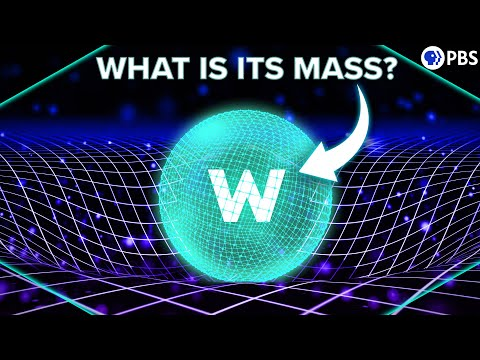### 2022-04-27: How the Higgs Mechanism Give Things Mass

• 01:35: ... the episodes   that led to this - fields and forces and  symmetries and all that. Similar to how   the fabric of a drum has ...
• 02:26: We saw in our episode on Noether’s theorem that  these symmetries lead to conservation laws.
• 03:09: ... one of the fundamental forces arises from  a symmetry of nature - in this case the fact   that the laws of physics ...
• 03:36: ... trick to explain the weak interaction   as arising from symmetries. We saw that we could  invent a pair of totally abstract degrees ...
• 04:08: ... deal breaker actually - massive bosons break gauge   symmetries. According to something called  Goldstone’s theorem, all gauge ...
• 04:37: ... so that they apply at the same time. We call this  combined symmetry group U(1)xSU(2). The resulting   gauge field still has bosons ...
• 05:24: ... defines electric charge.   Is it time to give up on this symmetry stuff ...
• 05:38: ... clue. I said  that massive bosons break the gauge   symmetry. But what if that’s okay? We’ve also  talked about the idea of ...
• 06:43: ... Now the current state of the system   has a broken symmetry, even if the  symmetry of the landscape ...
• 07:01: ... let’s just see if we can break  the symmetries of the universe   in a similar way. The equivalent of ...
• 09:00: ... within this field. This   particular Lagrangian has the simple symmetry that  it’s the same if you reflect it around the y ...
• 09:34: ... would be a global U(1) symmetry. Repeating  our electromagnetism trick means requiring ...
• 10:50: ... still a symmetric potential - it has the  same global U(1) symmetry as the original.   But if the universe suddenly transitions ...
• 11:02: ... correspond to different physical states.  We say that the symmetry is spontaneously  broken. The current state of the field ...
• 11:28: ... is just like how the field of magnets  can be in a state of broken symmetry   even though the overall equations  of magnetism have not ...
• 12:59: ... interactions, so we need simultaneous local  U(1) and SU(2) symmetry. Let’s just do U(1)   because that gives us the basic picture. ...
• 16:02: ... boson 10 years ago verified the idea that the   underlying symmetries of nature explain and  unify some of the forces of nature. ...### 2022-03-23: Where Is The Center of The Universe?

• 17:09: When the universe cooled down and the electroweak symmetry was broken, particles were locked in whatever isospin state they happened to be in.### 2022-03-16: What If Charge is NOT Fundamental?

• 07:27: He recognized that these patterns were actually representations of a mathematical symmetry known as SU(3).
• 07:34: ... we can’t get into the gory details of symmetry groups in this episode, but in short, Gell-Mann realized that he ...
• 12:43: That event - the breaking of electroweak symmetry  - created the weak and electromagnetic forces as we know them today.
• 13:24: We’ll find out soon as we continue to unravel the tangled symmetries of spacetime.### 2022-03-08: Is the Proxima System Our Best Hope For Another Earth?

• 19:27: ... unmeasurable - just the the phase of the wavefunction - it’s a symmetry of the Higgs field and doesn’t affect the behavior of the ...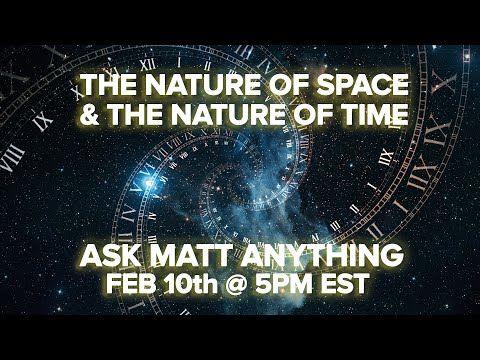### 2022-02-10: The Nature of Space and Time AMA

• 00:03: ... that their universe has a certain boundary condition that breaks the symmetry of time uh and that's simply that things were ordered in the past ...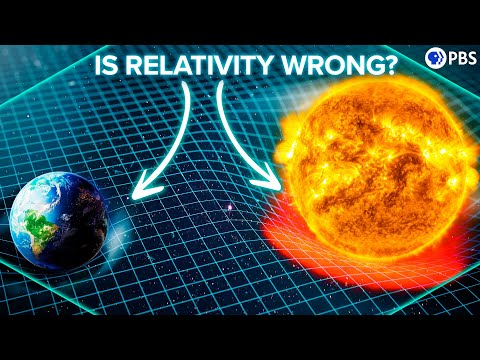### 2021-11-10: What If Our Understanding of Gravity Is Wrong?

• 16:02: In simple terms, the universe at its very core seems to be a set of symmetries which are manifest in the Lagrangian.
• 16:10: ... but we don't know all the symmetries  and we are not sure how those symmetries fit with each ...
• 16:23: It’s the symmetries of the Lagrangian via Noether’s theorem that yields our conservation laws and ultimately, well, all of physics.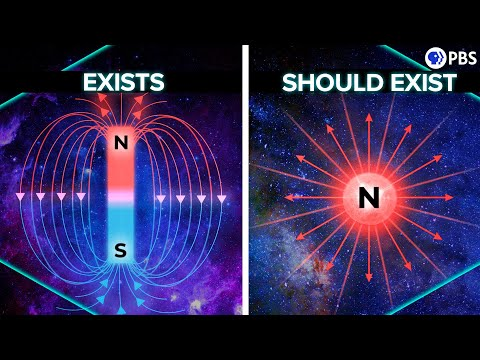### 2021-10-05: Why Magnetic Monopoles SHOULD Exist

• 03:30: ... a near perfect symmetry between electricity and magnetism which only gets screwed up when you ...
• 03:40: You could also have symmetry between these equations if there was such a thing as magnetic charge.
• 04:37: ... from requiring that the equations of quantum mechanics had a particular symmetry - the measurements they predict are unaltered by changes in one simple ...
• 09:00: We talked about that before - about how the breaking of the symmetry of the Higgs field separated the weak and electromagnetic forces.
• 09:16: These involved slightly more complicated symmetry breakings.
• 13:48: And so many of us remain obsessed with this elusive beast, and convinced of its inevitability according to the symmetries of space time.### 2021-09-21: How Electron Spin Makes Matter Possible

• 02:18: ... groups of particles that have two properties: 1) this weird rotational symmetry, and 2) indistinguishability - which is just the fact that all electrons, ...
• 14:26: ... are indistinguishable and they obey a simple, if odd, rotational symmetry. Ironically, to prove the structural integrity of matter I had to ...### 2021-09-15: Neutron Stars: The Most Extreme Objects in the Universe

• 04:39: ... each other, like gridlocked traffic. And the   symmetry of that repulsion forces nuclei into a regular grid. In this case ...### 2021-06-23: How Quantum Entanglement Creates Entropy

• 16:39: ... But we know for sure that this universe has a continuous rotational symmetry - there   is no preferred angle. That’s the basis for ...### 2021-04-21: The NEW Warp Drive Possibilities

• 09:43: That gave them an axial symmetry.### 2021-02-10: How Does Gravity Warp the Flow of Time?

• 07:22: But as soon as one of those observers changes direction, the symmetry is broken.### 2020-12-08: Why Do You Remember The Past But Not The Future?

• 07:50: But in the case of the asteroid, time symmetry is much more easily broken.
• 13:26: Yossi Sirote asks essentially the same question - doesn't the collapse of the wave function break time symmetry.
• 13:33: ... collapse is a random rather than deterministic event, then time-reversal symmetry is broken the arrow if time is established by quantum ...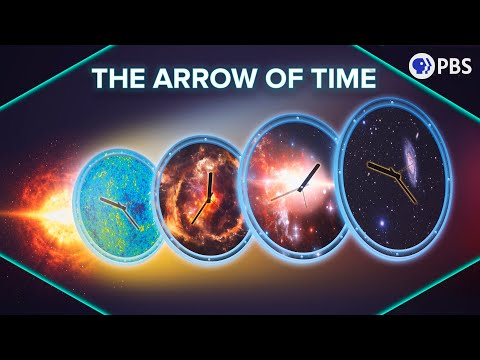### 2020-11-18: The Arrow of Time and How to Reverse It

• 01:21: ... going to dive into the first part of this - How does entropy break the symmetry of time, and cause the future to differ from the past. We’ll follow with ...
• 02:53: ... the relationship between time slices of our block universe. Time has a symmetry in the sense that the past and future aren’t differentiated by the laws ...
• 03:12: ... time. So the 2nd law does dictate a direction for time - it breaks the symmetry. To see why, we’ll need to review entropy. We’ve talked about it before, ...
• 08:11: ... block universe connected by the laws of physics, then we can break the symmetry in the direction of time if one of those slices has a very unusual ...### 2020-11-04: Electroweak Theory and the Origin of the Fundamental Forces

• 00:08: But some extremely subtle clues in nature have led us to believe that the forces of nature were once unified, ruled by a single, grand symmetry.
• 00:20: And how do the forces of nature arise from mathematical symmetries in the first place?
• 02:19: But Fermi’s model only worked at low energies, and neither it nor its successors explain why the weak interaction violates charge-parity symmetry.
• 02:56: QED is what we call a gauge theory - its force-carrying fields and particles arise from the symmetries of the quantum equations of motion.
• 04:28: At least assuming that the symmetries of gauge theory remain intact.
• 05:42: So there we go - we insisted on a symmetry - that the Schrodinger equation is invariant to changes in the local phase of the wavefunction.
• 05:54: So that is gauge theory in a nutshell: it explains a force by imposing symmetries on the equations of motion.
• 06:57: ... ‘1’ just means the symmetry transformations act on states which can be completely represented by ...
• 07:08: Imposing this U(1) symmetry on our equations of motion led us to something very real and physical - electromagnetism.
• 07:14: So it makes sense to see what other symmetries do.
• 07:36: ... if we require this transformation to now respect the SU(2) symmetry - for example requiring unitarity - we knock out 5 degrees of freedom ...
• 08:08: The fields and corresponding particles produced by the pure symmetries we described are fundamentally massless.
• 08:40: The perfect masslessness of these gauge fields and bosons is a direct consequence of the perfect symmetries from which they come.
• 08:48: Adding in mass screws up those symmetries.
• 09:01: In order for the weak force bosons to have mass, we have to willingly break the symmetry that gave us them in the first place.
• 09:16: ... for the equations of motion describing a system to have a particular symmetry - to not care about changes in some property - but at the same time, for ...
• 09:53: This represents the symmetry in our equations.
• 10:27: This is a state of broken symmetry.
• 10:39: We call this spontaneous symmetry breaking.
• 10:48: ... the equations of motion respect the symmetries that give us the necessary gauge field, but the physical system - the ...
• 11:11: What is this symmetry?
• 11:21: It turns out that both the weak force AND electromagnetism come from the breaking of a larger symmetry group.
• 11:29: And actually, that larger symmetry group is just the combination of our U(1) and SU(2) symmetries.
• 11:36: The combined SU(2)xU(1) symmetry is the electroweak field, and it has 4 massless bosons, like a well behaved symmetry should.
• 11:48: ... at low temperatures, this symmetry is spontaneously broken - leaving an independent, massless s(1) field ...
• 12:33: We just asked what would happen if the equations of motion governing nature had certain symmetries.
• 12:39: The very existence of those symmetries requires a family of fields and particles that we now observe in nature.
• 12:47: ... bosons their mass - we have to conclude that really these fields, these symmetries, are ultimately ...
• 13:01: ... - the electroweak unification set us on this grand quest to find deeper symmetries that could bring the strong nuclear force into the fold, allowing us to ...
• 13:19: ... and the complexity they enable, inevitable consequences of the broken symmetries of ...
• 15:07: In the case of the perfect spherical symmetry inside of a Schwarzschild black hole, all geodesics do indeed converge.### 2020-10-27: How The Penrose Singularity Theorem Predicts The End of Space Time

• 02:48: ... a perfect point. Surely any   tiny deviation from spherical symmetry would cause particles to miss each other at the last ...### 2020-09-21: Could Life Evolve Inside Stars?

• 03:23: ... technical term is “spontaneous symmetry breaking” - the same events that led to the appearance of the separate ...
• 06:02: ... example, there’s this scenario in this early universe symmetry-breaking stuff where, after the monopoles form, they split in two - into ...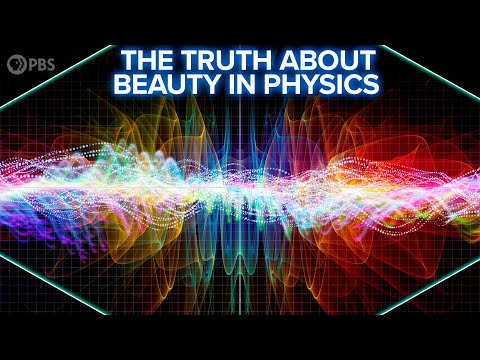### 2020-09-08: The Truth About Beauty in Physics

• 04:11: It’s the geometric symmetry of the circle or the platonic solid that seems beautiful.
• 04:17: ... in physics, when we talk about symmetry we mean that a physical law is unchanged by some transformation - ...
• 04:29: The universe does obey deep, underlying symmetries that are reflected on all scales of complexity.
• 04:36: ... make powerful use of these symmetries to derive our laws of physics, and so perhaps it’s not surprising these ...
• 10:13: Just add an additional degree of freedom - actually a symmetry - at each point in space and electromagnetism appears, almost miraculously.
• 11:30: His idea of introducing a new symmetry to space was translated to adding a new symmetry to the wavefunction in quantum mechanics.
• 11:43: ... Weyl’s idea evolved into what we now call gauge symmetry, and it’s the basis for the slightly ugly but fantastically successful ...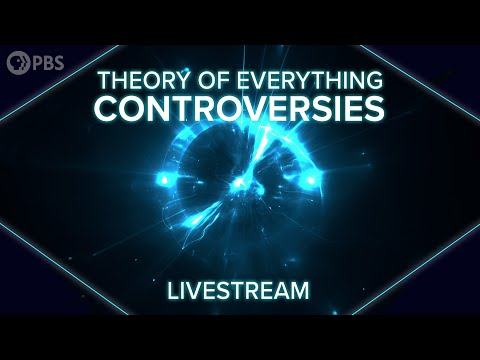### 2020-08-10: Theory of Everything Controversies: Livestream

• 00:00: ... particles and forces have the structure that they do the particular symmetries the particular arrangements of particles are like biologists before ...### 2020-07-28: What is a Theory of Everything: Livestream

• 00:00: ... is a principle of invariance the idea that there are underlying symmetries in this case symmetries that leave the speed of light um the same ...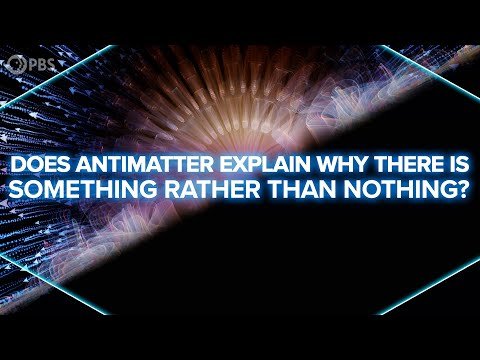### 2020-07-08: Does Antimatter Explain Why There's Something Rather Than Nothing?

• 02:12: ... many physicists think the answer lies in the fundamental symmetries of the universe, or, rather, in the breaking of these symmetries. We’ve ...
• 03:13: ... one by one, these presumed symmetries failed. The first to fall was parity, with Chien-Shiung Wu’s famous ...
• 04:05: ... maybe the violation of full CPT symmetry is needed to explain this imbalance. We saw in our previous episode that ...
• 04:48: ... of special relativity and the Standard Model are correct, then CPT symmetry must hold. But, we already know that our current understanding of the ...
• 07:21: ... regular hydrogen - deviations that could point to the violation of CPT symmetry. ALPHA uses CERN’s proton synchrotron to get their anti-protons. The ...
• 11:51: ... means we have a better idea of where to look for this strange BROKEN symmetry that leads to matter-antimatter imbalance and to the fact that we have ...### 2020-04-28: Space Time Livestream: Ask Matt Anything

• 00:00: ... a few hours but if it's something like gauge theory and electroweak symmetry breaking then working hard with the quantum physicist it takes us it ...### 2020-03-24: How Black Holes Spin Space Time

• 02:56: ... did it take so long? Well, general relativity is hard, and the spherical symmetry in the Schwarzschild solution eliminated a lot of complexity. Without ...### 2020-02-11: Are Axions Dark Matter?

• 00:03: ... does the strong nuclear force, the fundamental symmetries of nature, and a laundry detergent have in common? Well, they’re all ...
• 01:05: ... to go back and look at one of the most powerful concepts in physics: symmetry. We expect the laws of physics to be symmetric with respect to certain ...
• 02:35: ... of the strong force, derived with QCD, actually allow violation of CP symmetry. In fact they almost demand it - and yet no such violation has ever been ...
• 05:16: ... terms - the ones added by the weird vacuum - that appear to violate CP symmetry. That means the strong force should show CP violation. So why doesn’t it? ...
• 06:32: ... the strong CP problem its's that if any of the quarks are massless, CP symmetry is automatically conserved. However, as far as we can tell, none of the ...### 2020-01-27: Hacking the Nature of Reality

• 07:23: But in order to avoid those sums of Feynman diagrams, S-matrix theory also relies on symmetries between those virtual interactions.
• 07:32: In particular something called crossing symmetry.
• 07:49: And here’s another example of crossing symmetry.
• 09:11: ... based on these global consistency conditions and taking advantage of symmetries is also called a bootstrap model - from expression “pull yourself up by ...### 2019-10-07: Black Hole Harmonics

• 13:35: You can Learn probabilistic basics, such as fairness, expected value, and symmetry, in their course Probability Fundamentals.### 2019-04-10: The Holographic Universe Explained

• 06:25: By making this a conformal field theory we’ve added a symmetry –invariance under local changes in scale – also known as Weyl invariance.### 2019-04-03: The Edge of an Infinite Universe

• 16:39: In fact conservation of energy comes from this symmetry, as revealed by Noether's theorem.### 2019-01-24: The Crisis in Cosmology

• 13:32: Then we'll get to comments on our CPT symmetry episode.
• 15:08: Okay, let's move on to our episode on the ultimate symmetry of nature,...
• 15:22: The T in CPT symmetry isn't a literal rewinding of the clock.
• 15:36: If the universe has this sort of T symmetry,...
• 16:09: TinyFox Tom asks whether mass would be inverted under CPT symmetry.
• 17:13: As to the motion reversal symmetry of the T in CPT,...
• 17:23: But, at any rate, remember that T symmetry is broken.
• 17:27: Both the T of CPT and true time reversal symmetry.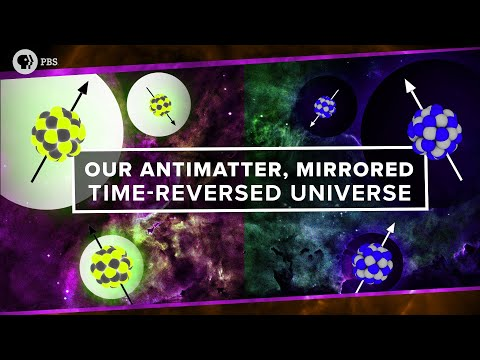### 2019-01-16: Our Antimatter, Mirrored, Time-Reversed Universe

• 00:03: ... foundations of quantum theory rest on its symmetries for example it should be impossible to distinguish our universe from one ...
• 02:02: ... behaves differently so what's the big deal well the violation of parity symmetry poses a threat to an even deeper symmetry cpt symmetry the combined ...
• 03:02: ... said right to left and send matter to antimatter at first glance this CP symmetry appears to hold not just in an imaginary clocks but also in the ...
• 08:43: ... here's the issue if CP symmetry is violated and CPT symmetry holds then T symmetry must also be violated ...
• 10:54: ... end up back in its starting configuration. On the other hand broken T symmetry says that if you do this reversal the future won't perfectly mirror the ...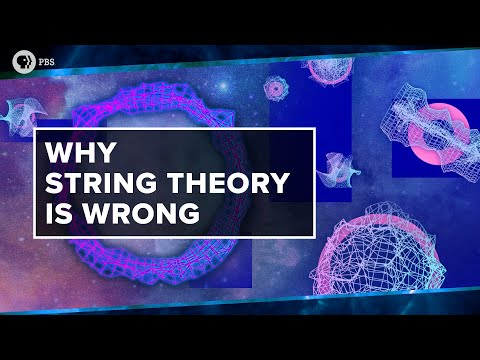### 2018-12-20: Why String Theory is Wrong

• 00:41: ... very first gauge symmetry -- Weyl invariance Einstein pointed out that the proposal led to some ...
• 05:30: ... is a theoretical symmetry between bosons and fermions, which very elegantly explains certain ...
• 05:47: ... introduction of supersymmetry along with the discovery of the right symmetries for the extra dimensions sparked the first superstring revolution of the ...
• 06:32: What differs is the detailed geometries and symmetries of those spaces, and the way strings vibrate within them.
• 14:34: ... idea of explaining electromagnetism by adding his gauge symmetry to general relativity was wrong, but it inspired the entire field of ...
• 14:48: It also gave us the sought after quantum electromagnetism in the end, just with a slightly different symmetry.### 2018-12-12: Quantum Physics in a Mirror Universe

• 00:02: ... at a universe whose laws are fundamentally different when we think about symmetry the first thing that comes to mind is mirror symmetry butterflies ...### 2018-11-14: Supersymmetric Particle Found?

• 01:46: ... a very natural explanation for the discrepancy by introducing a new symmetry between the fermions, which comprise matter, and the bosons, which ...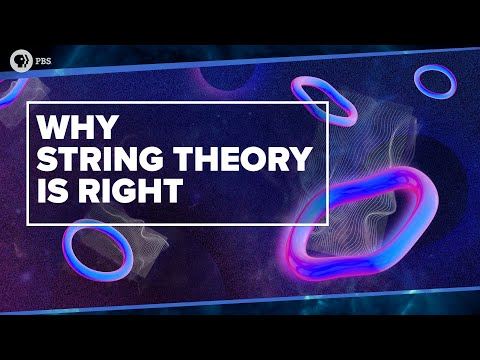### 2018-11-07: Why String Theory is Right

• 07:25: A big part of it is making use of symmetries.
• 07:28: ... particular coordinates or quantities, we say that that parameter is a symmetry of the system or that the system is invariant to transformations in that ...
• 07:42: Finding symmetries can massively reduce the complexity of the math.
• 07:46: A really important type of symmetry in quantum mechanics is gauge symmetry.
• 07:59: I want to remind you of one particularly crazy result of gauge symmetries.
• 08:11: So, we expect the phase of the quantum wave function to be a gauge symmetry of any quantum theory.
• 08:54: So in a way, electromagnetism was discovered in its quantum form by studying the symmetries of quantum mechanics.
• 09:02: ... turns out that exploring a very different symmetry of string theory both makes it possible to quantize the theory and gives ...
• 09:20: Applying the usual old symmetries got physicists some of the way, but to succeed, they needed an extra weird type of symmetry.
• 09:29: That symmetry is Weyl symmetry or Weyl invariance.
• 09:43: Hermann Weyl actually came up with this symmetry right after Einstein proposed his general theory of relativity.
• 09:54: ... story-- Weyl invented the name gauge symmetry to describe this scale invariance inspired by the gauge of railroad ...
• 10:06: Anyway, Weyl symmetry doesn't work.
• 10:30: ... the 2D sheet traced out in spacetime by a vibrating 1D string has this symmetry that lets us redefine the scale on its surface however we ...
• 11:04: But there's a cost to using this symmetry.### 2018-10-25: Will We Ever Find Alien Life?

• 13:32: Now, this is a type of gauge symmetry that, in principle, unifies fermions and bosons.
• 13:42: Adding the symmetry gives you fermions but it also shaves off dimensions.### 2018-07-11: Quantum Invariance & The Origin of The Standard Model

• 00:15: But even more stunning is how it was discovered, by peering deep into the symmetries of reality.
• 00:38: Previously, we've talked a bit about the symmetries of these equations and how they lead us to conserved quantities like energy and momentum.
• 00:49: These symmetries can be portals into entirely new aspects of reality.
• 01:47: It's what we call a gauge freedom or a gauge symmetry.
• 01:57: But it turns out that these gauge symmetries are an important feature of most of our physical theories describing the universe.
• 02:16: A theory that has these gauge symmetries is called a gauge theory.
• 02:20: Today, we're going to look at the simplest of the symmetries of the standard model.
• 03:29: And this innocuous seeming step introduces a simple symmetry that has profound implications.
• 05:02: Global phase is a gauge symmetry of the system.
• 05:05: Let's push a little further to see how far this symmetry goes.
• 06:26: Local phase is not a gauge symmetry of the basic Schrodinger equation.
• 07:52: We just rediscovered electromagnetism by insisting on a gauge symmetry that we had no right to expect to exist in the first place.
• 08:12: And now we know how it interacts with particles of matter to give them this symmetry.
• 08:40: By the way, applying Noether's theorem tells us there is a conserved quantity associated with any symmetry.
• 08:48: In this case, the symmetry is local phase invariance and the conserved quantity is electric charge.
• 09:35: To understand those, we'll need to go beyond the Schrodinger equation and to explore new gauge symmetries.
• 09:41: It turns out that local phase invariance is just the simplest of the larger suite of gauge symmetries of the standard model.
• 09:49: ... symmetries are obtusely named, U1, SU2 and SU3, and they predict the fields that ...
• 10:02: ... fields that arise from these gauge symmetries are called gauge fields, and they all have their associated ...
• 10:29: ... here is not the nature of the quantum field nor the connection between symmetry and the fundamental forces, perhaps it's the fact that by pure ...### 2018-05-23: Why Quantum Information is Never Destroyed

• 01:07: And yet, this sort of deterministic symmetry, time-reversal symmetry, is essential for information itself to be conserved.
• 01:34: ... to discover quantities that are conserved in a system by looking at the symmetries of the equations of ...
• 01:49: ... theorem applies to smooth, continuous symmetries where we can transform the chosen coordinate, however much we like, ...
• 01:58: But there are also symmetries under discrete transformations.
• 02:13: Symmetry in that last one.
• 02:14: Time-reversal symmetry isn't covered by Noether's theorem, but it's still tied to a conservation law.
• 02:57: ... one thing, this time-reversal symmetry means complete information about the configuration at all prior times ...
• 04:36: It ensures conservation of information and time-reversal symmetry because of an even more fundamental rule-- the conservation of probability.
• 06:56: ... how probability works, but the condition also ensures time-reversal symmetry and conservation of ...
• 11:03: Noether's theorem predicts the conservation laws of physics from the symmetries of nature.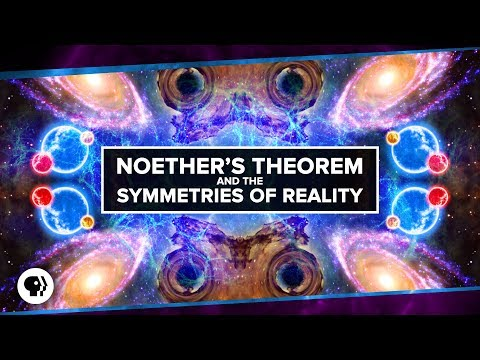### 2018-05-16: Noether's Theorem and The Symmetries of Reality

• 00:46: They emerge from the fundamental symmetries of nature.
• 00:49: The connection between conservation laws and symmetry is encapsulated in Noether's theorem.
• 02:08: For every continuous symmetry of the universe, there exists a conserved quantity.
• 02:14: First, what do we mean by symmetry?
• 02:29: But these are what we call discrete symmetries-- single flips around one axis or rotation by specific amounts.
• 02:36: Noether's theorem applies to continuous symmetries.
• 03:17: We have symmetry to spatial translation.
• 04:01: Noether's theorem reveals that this time translation symmetry gives us energy conservation.
• 04:43: If the very nature of space can change over time, then continuous time symmetry is broken.
• 07:15: As long as we can identify that system's symmetries, this is useful in cosmology, but it's also useful in quantum physics.
• 07:22: ... general nature of the theorem means we can apply it to not just the symmetries in the dimensions of space and time, but also to more abstract ...
• 07:36: Noether's theorem tells us that there should be a corresponding symmetry of nature, and there is.
• 07:42: That symmetry is the phase of the quantum field.
• 07:54: This symmetry leads to the conservation of electric charge and electric current.
• 07:59: This quantum symmetry is just the simplest of a large number of symmetries exhibited by quantum fields, the so-called gauge symmetries.
• 08:26: It's founded on the fundamental symmetries of quantum fields.
• 08:30: ... going to take a few episodes to explain exactly what these symmetries really are and how they lead to the family of particles and interactions ...
• 09:27: ... the fundamental workings of the universe through the continuous symmetries of "Space Time." Thank you to Brilliant for supporting PBS Digital ...### 2018-02-28: The Trebuchet Challenge

• 02:53: This fact gives us insight into the fundamental symmetries of nature.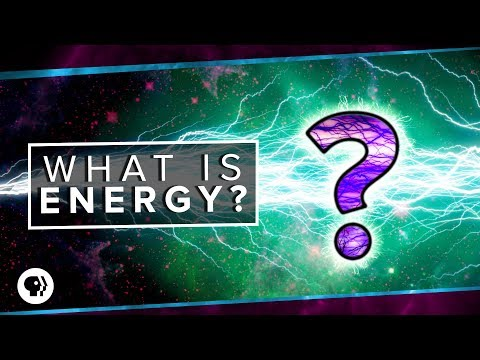### 2018-02-14: What is Energy?

• 09:45: See, the law of conservation of energy arises because of symmetry, in particular time translational symmetry.
• 10:00: In fact, for every symmetry in our universe, there exists a conserved quantity.
• 10:06: For example, the law of conservation of momentum is due to spatial translation symmetry.
• 10:16: ... relationship between conservation laws and symmetries was discovered by mathematician Emmy Noether and Noether's theorem is ...### 2017-11-02: The Vacuum Catastrophe

• 07:59: ... high vacuum energy or a vacuum energy of exactly 0 if we assume symmetry of positive and negative zero points between different fields, but a ...
• 08:28: That sounds like a ridiculously unlikely bit of luck if we can't invoke symmetries.### 2017-08-10: The One-Electron Universe

• 06:16: In the sense of these fundamental symmetries, anti-matter is time-reversed matter.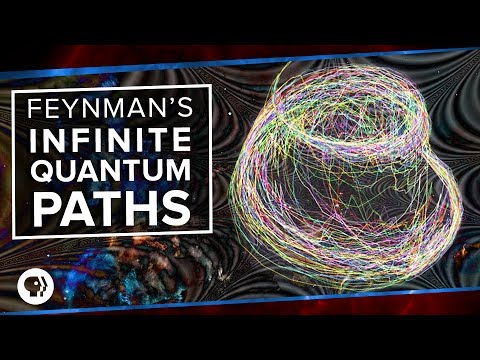### 2017-07-07: Feynman's Infinite Quantum Paths

• 14:38: It indicates a break in what we once thought to be a fundamental symmetry of the universe.### 2017-03-15: Time Crystals!

• 02:01: Wilczek came up with a simple model in which charged particles in a superconducting ring break what we call continuous time translational symmetry.
• 02:34: ... imaginary system broke this time translational symmetry, because there are global statistical differences in the state of the ...
• 03:15: This would break time translational symmetry, which makes most physicists nervous.
• 03:23: ... of Tokyo showed from theoretical arguments that time translational symmetry can't be broken by a quantum system in ...
• 08:07: ... also add that while their systems do break continuous time translational symmetry, they have a different type of symmetry-- discrete time ...
• 09:15: Before this year, time stood out as a major symmetry that hadn't been broken.### 2016-03-16: Why is the Earth Round and the Milky Way Flat?

• 00:44: Two key principles, equilibrium and symmetry.
• 01:33: And it's the symmetries of the forces at work in creating that equilibrium that decide what that final shape will be.
• 01:41: So let's talk about symmetry.
• 01:43: ... terms of shape, things like planets and stars have spherical symmetry, meaning you can rotate them in three dimensions and the basic shape ...
• 01:53: disk-shaped things like galaxies and solar systems have circular symmetry.
• 02:04: But how does a force have symmetry?
• 02:34: ... gravity has spherical symmetry in the sense that, if you're some distance from a space thing of any ...
• 04:56: See, pressure shares that same symmetry as gravity.
• 08:36: It's because the effect that prevents their collapse has a very different symmetry.
• 10:14: Now, these fundamental symmetries don't just define the shapes of some of the largest things in our universe.
• 10:34: Symmetries really do shape the universe on all the scales of space time.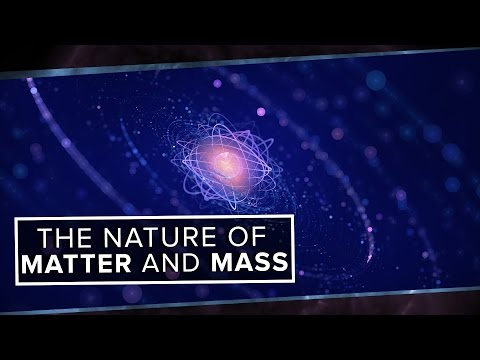### 2016-01-06: The True Nature of Matter and Mass

• 10:17: Only when the universe cooled down did the Higgs field gain a nonzero value in a phenomenon called spontaneous symmetry breaking.### 2015-10-07: The Speed of Light is NOT About Light

• 07:05: It's the only one that satisfies all of these pretty fundamental statements about the relativity, symmetry, and consistency of our universe.
• 07:40: And honestly, just from the symmetry and relativity arguments that we made, c could be infinity.
51 result(s) shown.